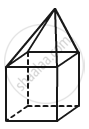Advertisement Remove all ads

# Verify Euler'S Formula for the Following Polyhedron: - Mathematics

Verify Euler's formula for the following polyhedron:Advertisement Remove all ads

#### Solution

In the given polyhedron:

Edges E=16

Faces F=9

Vertices V=9Now, putting these values in Euler's formula:

RHS: F+V

= 9+9

= 18

LHS: E + 2

= 16 + 2

= 18

LHS = RHS

Hence, Euler's formula is satisfied.

Is there an error in this question or solution?
Advertisement Remove all ads

#### APPEARS IN

RD Sharma Class 8 Maths
Chapter 19 Visualising Shapes
Exercise 19.1 | Q 6.2 | Page 9
Advertisement Remove all ads
Advertisement Remove all ads
Share
Notifications

View all notifications

Forgot password?
Course